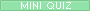studentteacher Ms. Carroll Grayson County Middle School math/social studies Send e-mailHello to all I am thrilled that I figured out how to do this.  Hope you all enjoy doing a math assignment and quiz on the computer!  Since we have been discussing connecting math to the real world, you should have some background information on the history of math.  After doing the following activitiy, there is a math quiz for you to take.       You are to select the sites listed below to define each of these branches of mathematics: Arithmetic- Algebra- Geometry analytic geometry Trigonometry Calculus Analysis Probability Statistics Set theory logic Now, use the site below to help you with your math quiz.
My Quia activities and quizzesmath history quiz
https://www.quia.com/jq/12919.html
quiz on branches of math